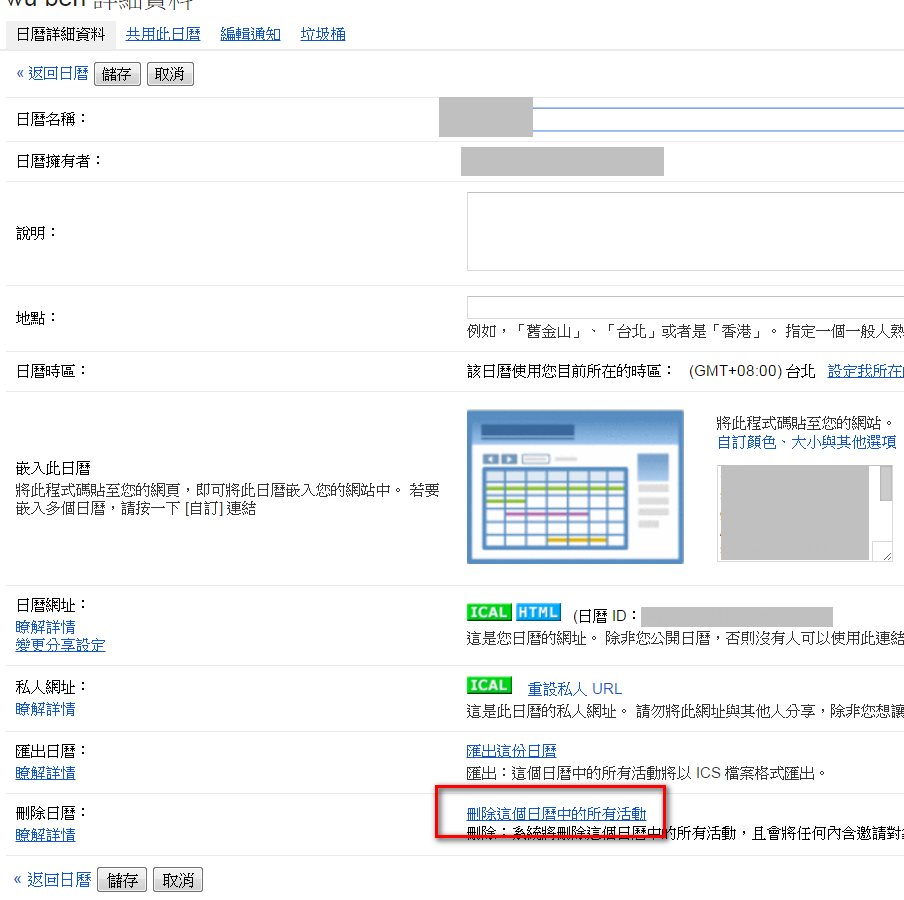#7

## 在 Google Calendar 上刪除所有活動``````/**
* 開啟 Spreadsheet 的時候新增 Menu
*/
function onOpen(e) {
//選單名稱
var m = SpreadsheetApp.getUi().createMenu('Google Calendar Functions');
//選單項目
m.addItem('get calendar ids', 'getids');
m.addItem('delete all calendar envents', 'delAllCalEvent');
m.addToUi();
}
/**
* 取得所有日曆
*/
function getids() {
//取得目前所在的工作表
var sht = SpreadsheetApp.getActiveSheet();
//清除工作表內容
sht.clear();
//取得所有日曆
var ary = CalendarApp.getAllOwnedCalendars();
if (ary.length === 0) {
sht.getRange(1, 1).setValue("找不到已存在的日曆");
return;
}
//設定標題
sht.getRange(1, 1, 1, 2).setValues([["ID", "名稱"]]);
//顯示所有日曆
for (var i=0; i<ary.length; i++){
sht.getRange(i + 2, 1, 1, 2).setValues([[ary[i].getId(), ary[i].getName()]]);
}
//設定欲刪除的日曆輸入框
sht.getRange(ary.length + 2, 1).setValue("請輸入欲刪除的日曆 ID:");
}
/**
* 刪除指定日曆下的所有事件
*/
function delAllCalEnvent(){
//取得目前所在的工作表
var sht = SpreadsheetApp.getActiveSheet();
var lastrow = sht.getLastRow();
var idRow = 0;
for (var j=1; j<lastrow; j++){
if (sht.getRange(j, 1).getValue() == "請輸入欲刪除的日曆 ID:"){
idRow = j;
}
}
if (idRow > 0){
//取得欲刪除的日曆 ID
var calid = sht.getRange(idRow, 2).getValue();
//取得該日曆物件
var cal = CalendarApp.getCalendarById(calid);
if (cal != null){
//取得該日曆下從 1990/01/01 到 2078/12/31 期間所有的事件
var ary = cal.getEvents(new Date("1990/01/01"), new Date("2078/12/31"));
for (var i=0; i<ary.length; i++){
sht.getRange(idRow + i + 1, 1).setValue(ary[i].getTitle());
ary[i].deleteEvent();
sht.getRange(idRow + i + 1, 2).setValue("已刪除");
}
}
}
}

``````

1. get calendar ids : 點選這個選項會取得你的所有日曆並顯示在工作表中，其中的 ID 是 Google Calendar 內部的識別碼，要靠這個才知道要刪哪個日曆的活動
2. delete all calendar envents: 必須先在工作表中的 "請輸入欲刪除的日曆 ID:" 後一欄輸入上一個功能取得的ID，再點選此選項就可以刪除活動了!

0